# Orthogonal projection of a Plane

### Traces of a Plane

Beside the three principal planes of projection (Π1, Π2, Π3), there are three types of planes in special positions:

• the 1st projecting plane or a horizontal projecting plane – a plane perpendicular to Π1,
• the 2nd projecting plane or a vertical projecting plane – a plane perpendicular to Π2,
• the 3rd projecting plane or a profile projecting plane – a plane perpendicular to Π3.

If a plane is none of these types, it is in general position with respect to Π1, Π2 and Π3,i.e. its image to all of these three projections is entire plane of projection.
Therefore, if we project a plane, we distinguish its special lines, the intersecting lines with three projection planes which are called the traces of the plane. Planes will be denoted by upper Greek letters, and the traces by corresponding small Latin letters (as an example: A ↔ a, B ↔ b, Γ ↔ g, Δ ↔ d, P ↔ r, Σ ↔ s).

Let Ρ be a plane in general position. Then:

• the line r1 = PΠ1 is called the 1st trace or horizontal trace, (r1'' = x, r1''' = y),

• the line r2 = PΠ2 is called the 2nd trace or vertical trace, (r2' = x, r2''' = z),

• the line r3 = PΠ3 is called the 3rd  trace or profile trace, (r3' = y, r3'' = z).

### Defining a plane by three numbers

Any plane that does not contain the origin O(x,y,z) of the coordinate system, it is intersected by the coordinate axes in three points:

X = x ∩ P, the intersection point of the axis x and the plane P,
Y = y ∩ P, the intersection point of the axis y and the plane P,
Z = z ∩ P, the intersection point of the axis z and the plane P.

Let us denote the three intersection points:
X = (ξ,0,0),Y = (0,η,0),Z = (ζ,0,0),
then it is clear that the three numbers (ξ,η,ζ) determine uniquely the position of a plane in the space as well as its traces. It is shown in the figure below.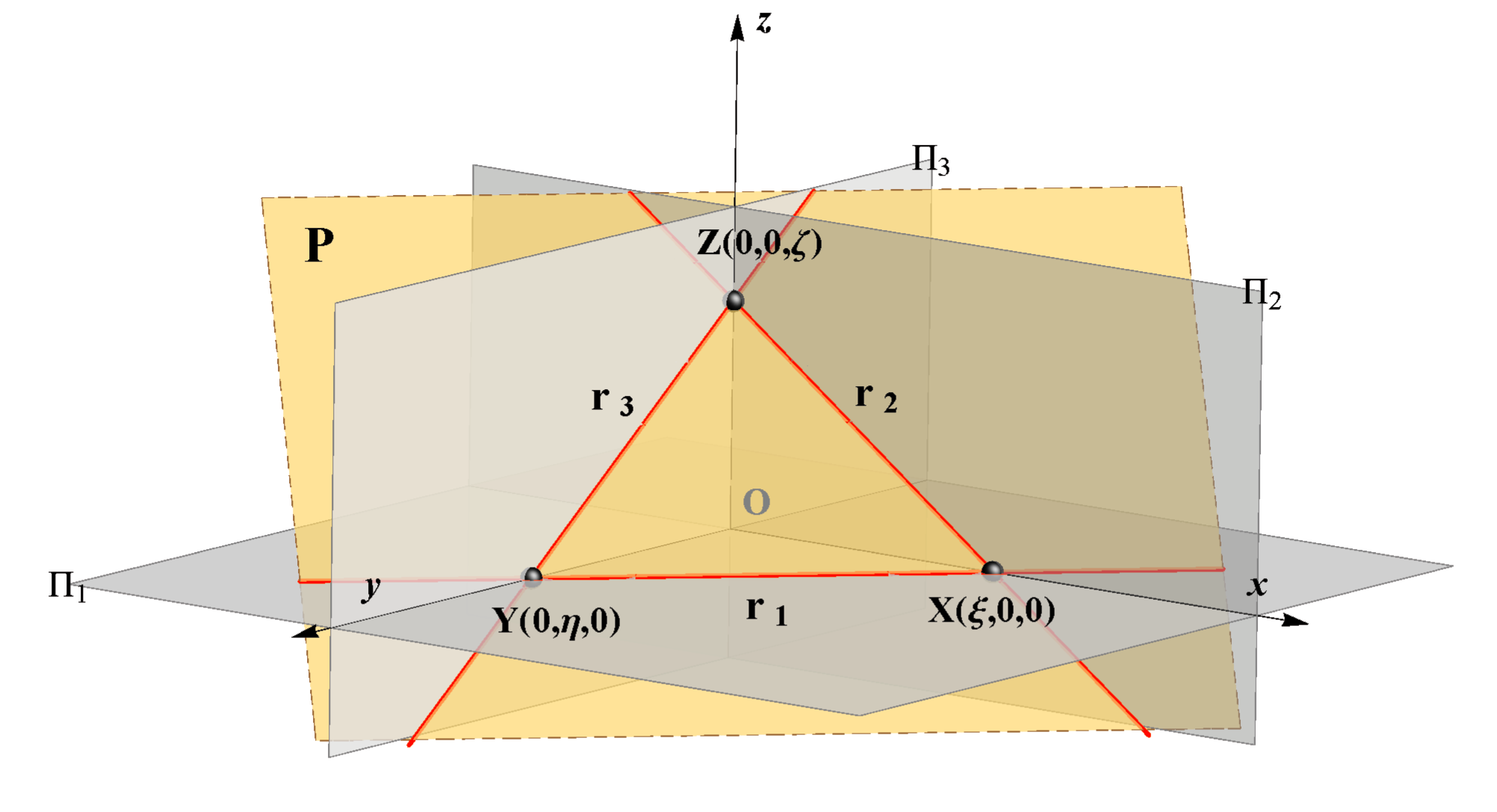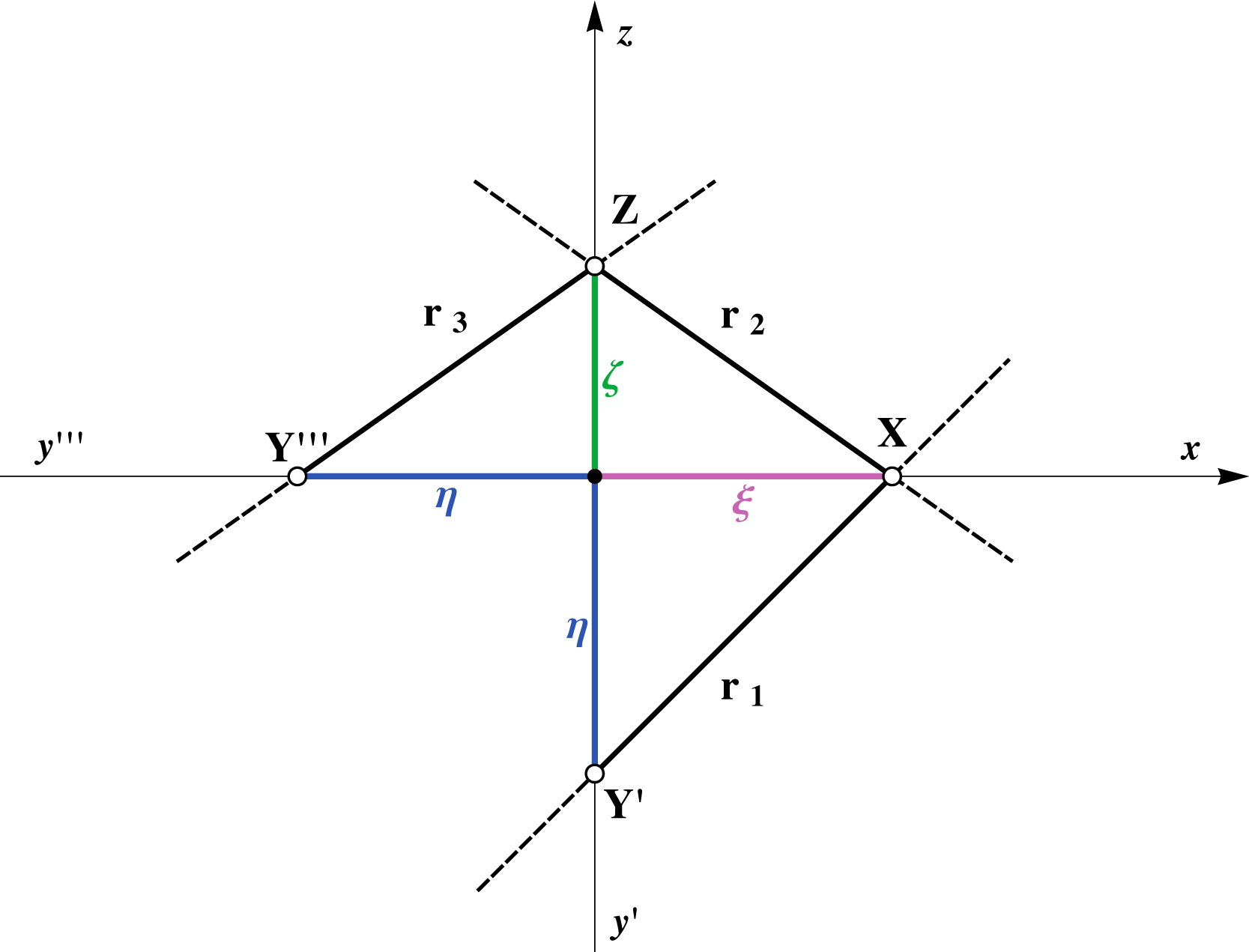Every pair of traces must intersect in a point lying on one coordinate axis:

r1 ∩ r2 = X ∈ x,r1 ∩ r3 = Y ∈ y,r2 ∩ r3 = Z ∈ z.

In the figure above we can see how to denote (the notation of) the traces. If all three traces are drawn, they are drawn as a solid line only on the part which lies in the  I. octant. Other parts, if necessary, are drawn as dashed lines.
This means that the horizontal trace is drawn as a solid line beneath the x axis and on the right  side of y' axis.

Assignment 1: Construct and label the traces of the following planes: A(4,2,3), B(3,2,–4), Γ(4,2,), Δ(4,,2) and E(,4,2).

We will mostly draw only the horizontal and vertical traces. If it is so, they will be drawn as a solid line in the following way: the horizontal trace is the solid line beneath the x axis, and the vertical trace is the solid line above the x axis.

Assignment 2: Construct and label the horizontal and vertical traces of the following planes:
Σ(–4,2,5), Κ(–4,–2,5), Ω(–4,–2,–5), Λ(–4,–2,), Φ(–4,,–5), Ψ(,–2,–5), Ζ(,,2), Τ(,2,) and Θ(2,,).

### Visualization of the change of the traces

The following animation shows how the vertical and profile traces change during the rotation of the plane about its horizontal trace. Vertical and profile traces always intersect in a point lying on the z axis.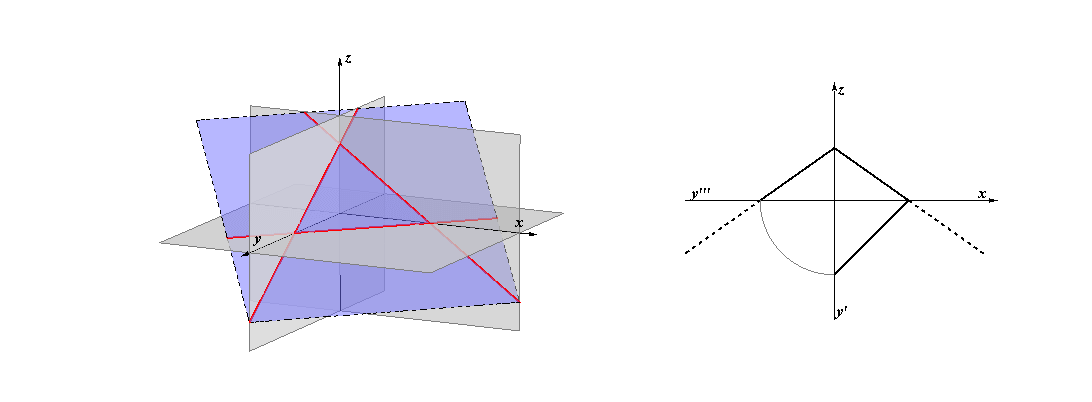Animation starts by clicking in the figure above.

### Planes in special positions

##### Projecting planes
Planes perpendicular to at least one plane of projection are parallel to at least one coordinate axis. Therefore, their image in that projection is a line - corresponding trace.

 horizontal projecting plane E E ⊥ Π1, E || z e1 = E' vertical projecting plane E E ⊥ Π2, E || y e2 = E'' profile projecting plane E E ⊥ Π3, E || x e3 = E'''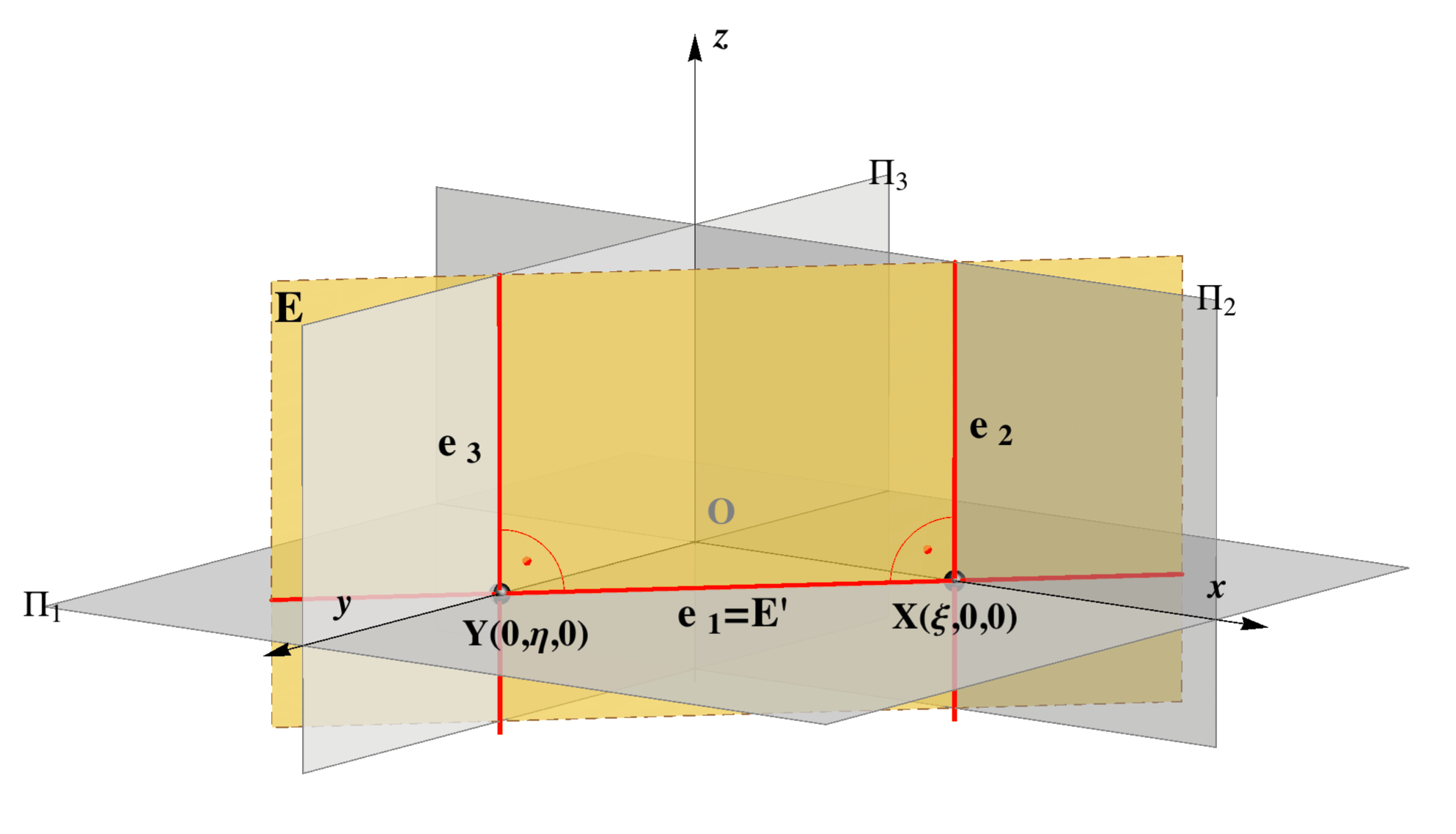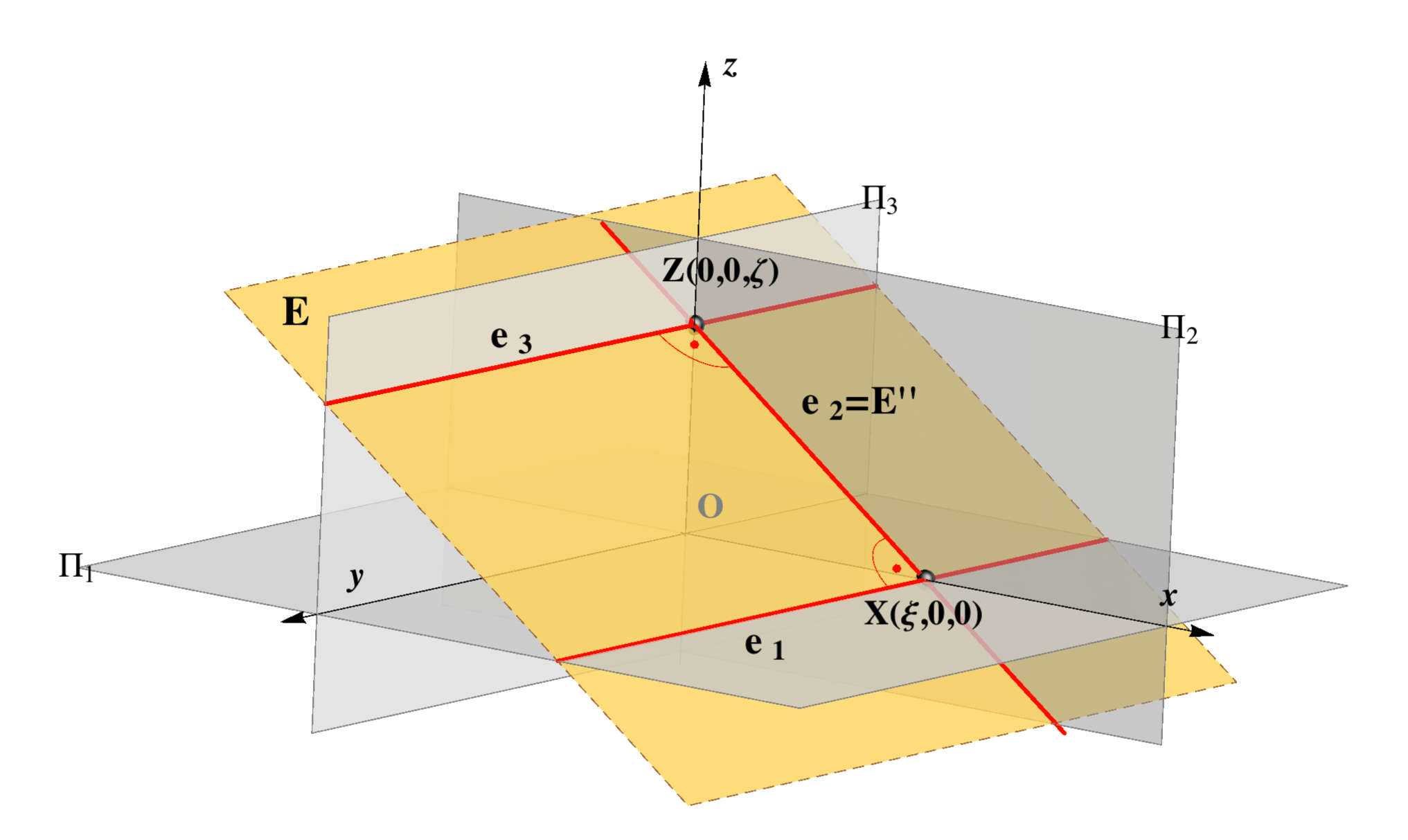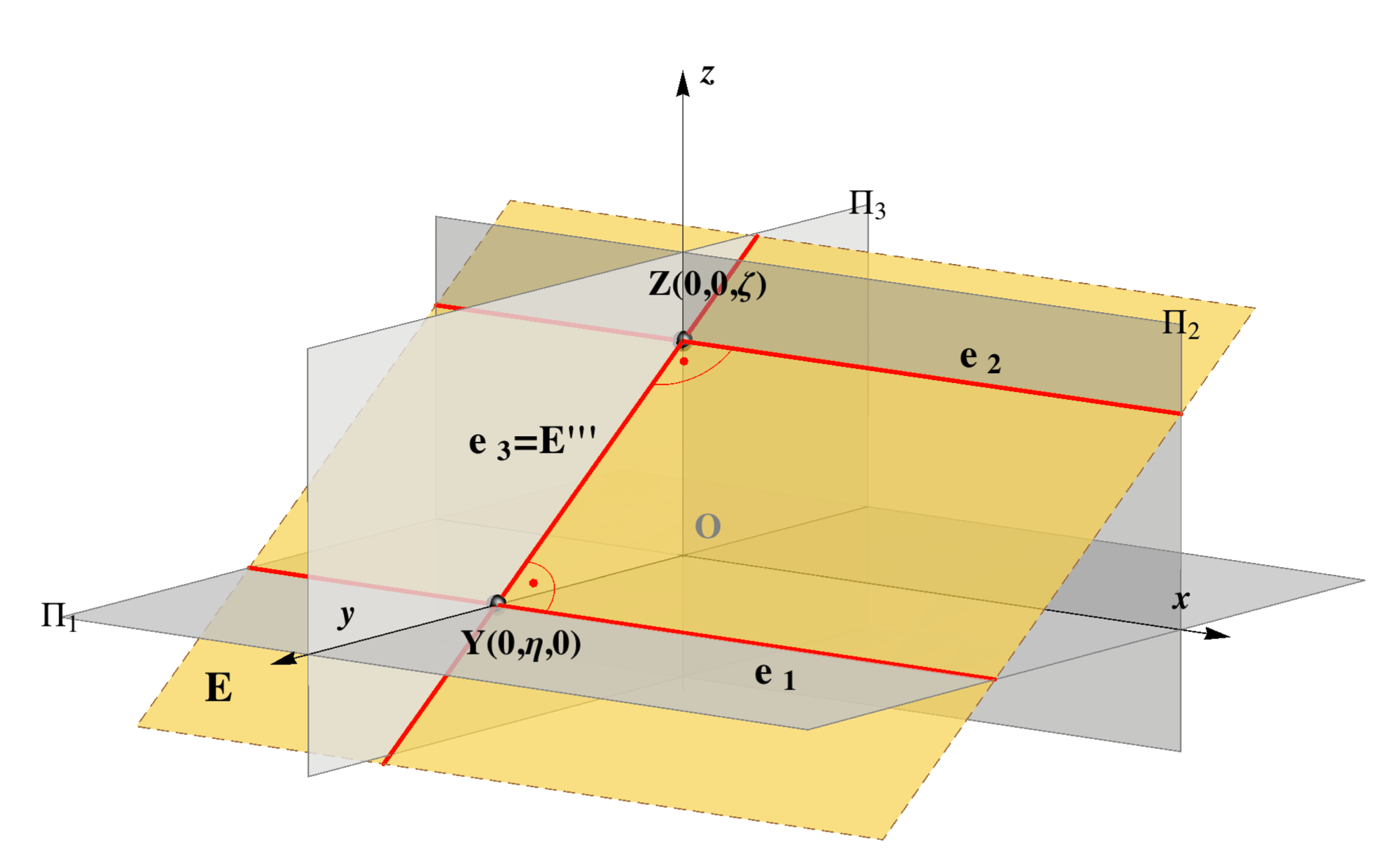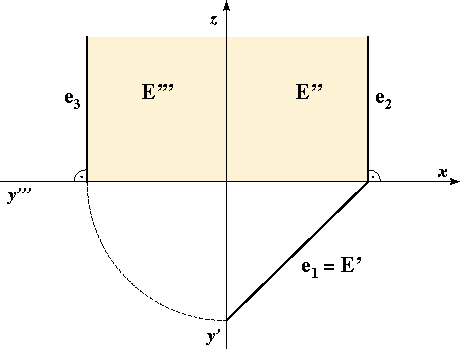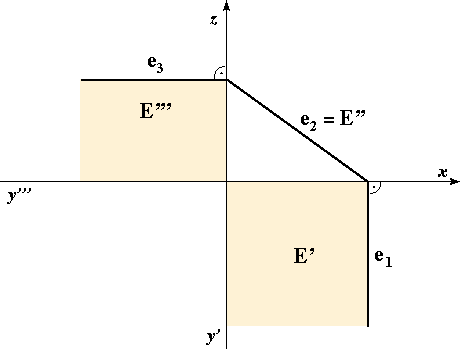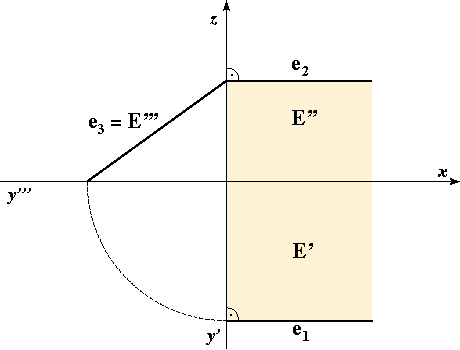Σ || Π1, Σ ⊥ z Σ ⊥ Π2, Π3 Σ || x, y Σ || Π2, Σ ⊥ y Σ ⊥ Π1, Π3 Σ || x, z Σ || Π3, Σ ⊥ x Σ ⊥ Π1, Π2 Σ || y, z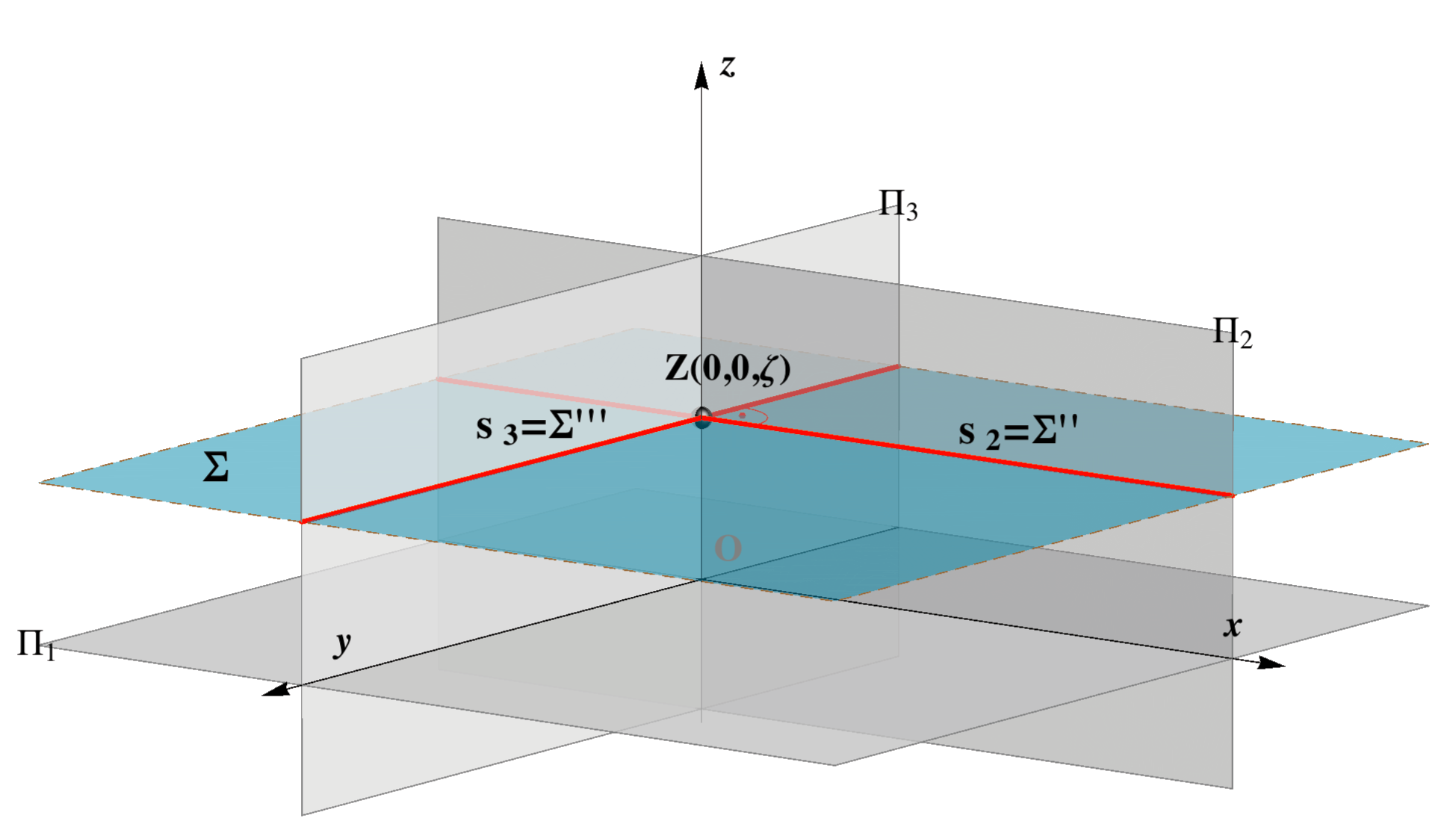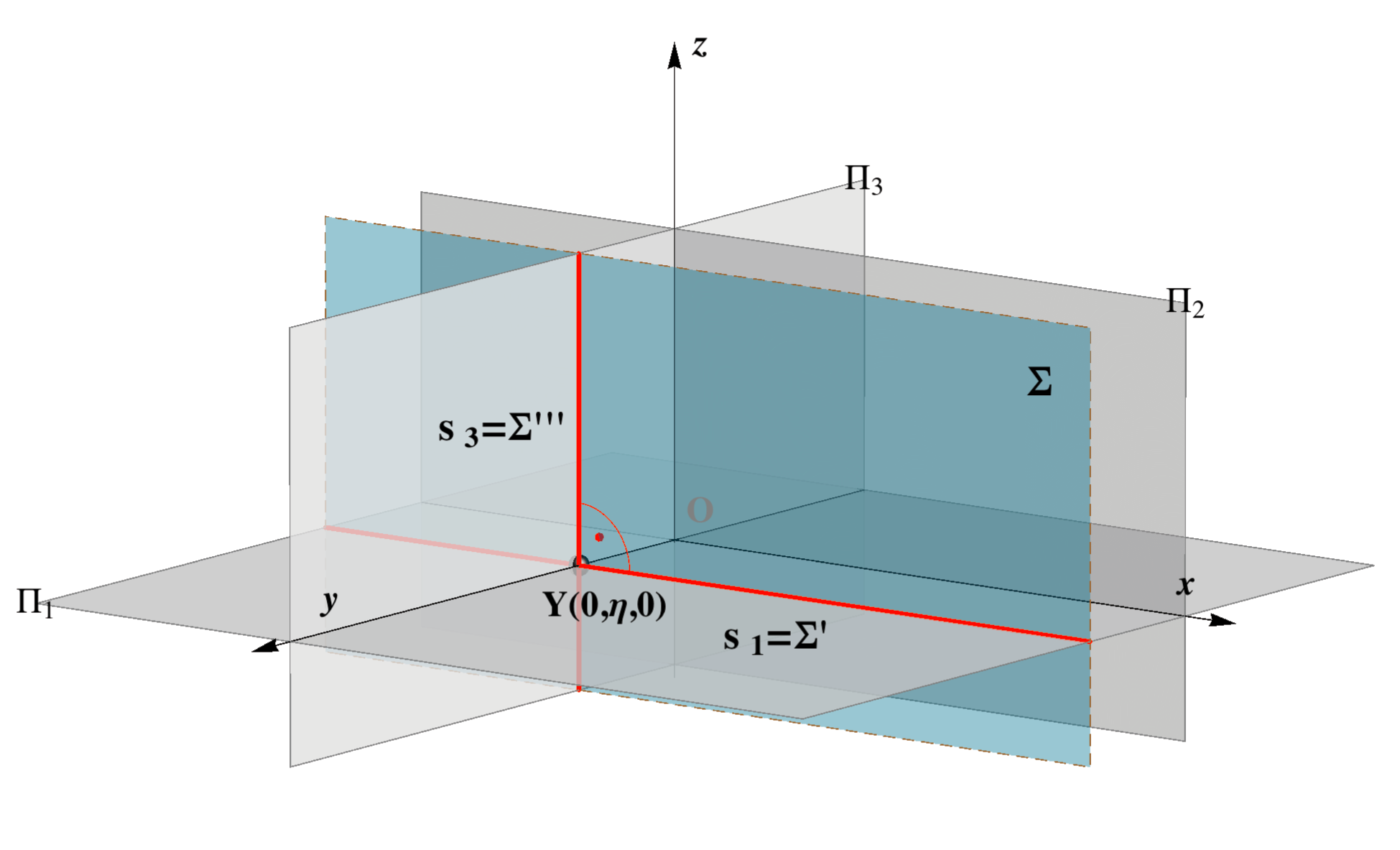Trace s1 is the line at infinity of the plane Π1. This type of planes are called horizontal planes. Trace s2 is the line at infinity of the plane Π2. This type of planes are called vertical planes. Trace s3 is the line at infinity of the plane Π3. This type of planes are called profile planes.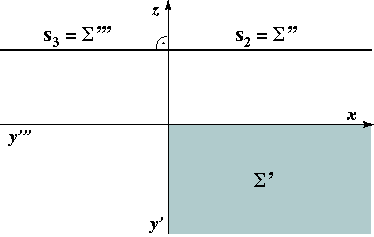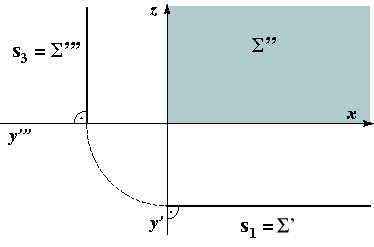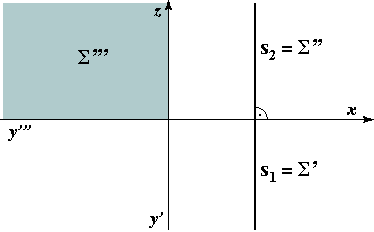On these image we highlight the areas where the points of the plane that lie in the 1. octant are projected.

#### Planes that contain the origin O

Traces of these planes are not uniquely determined by the triplet of numbers  (ξ,η,ζ) .
For instance, Ρ(0,0,0) indicates only that  Ρ contains the origin, Ρ(,0,0), Ρ(0,,0) or Ρ(0,0,) indicate that the plane contain the axis x, y or z.
In this situation, we require more data on the plane.

• The plane contains one of the axis and this axis coincide with two traces of the plane.
This plane is perpendicular to the projecting plane whose trace we don't know. It is enough to give one point of the plane that lies outside of the axis. The projection of that point determines the unknown trace.

Examples of this kind of planes are the symmetry plane and the coincidence plane. Their traces are shown in the figure below.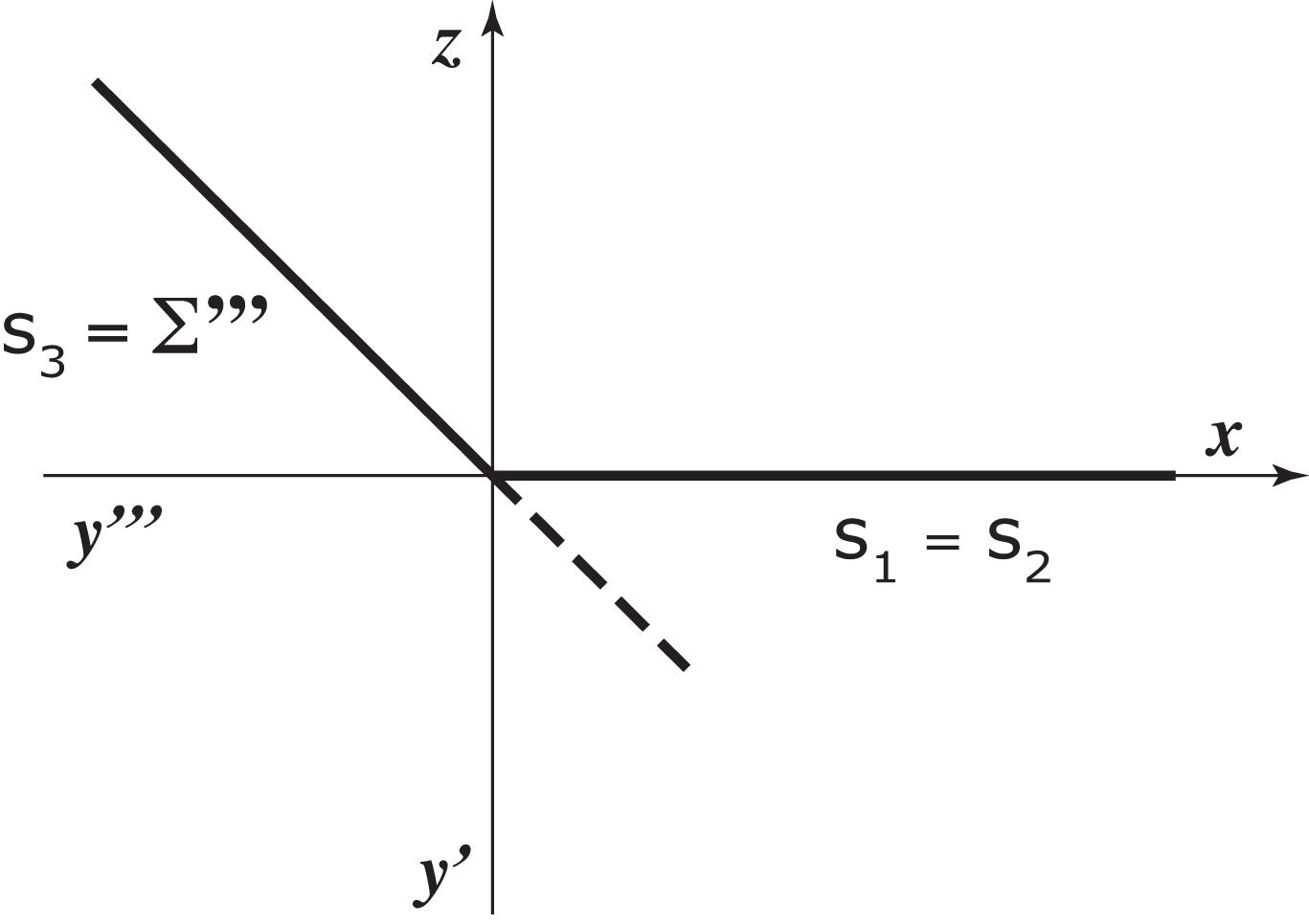Traces of the symmetry plane.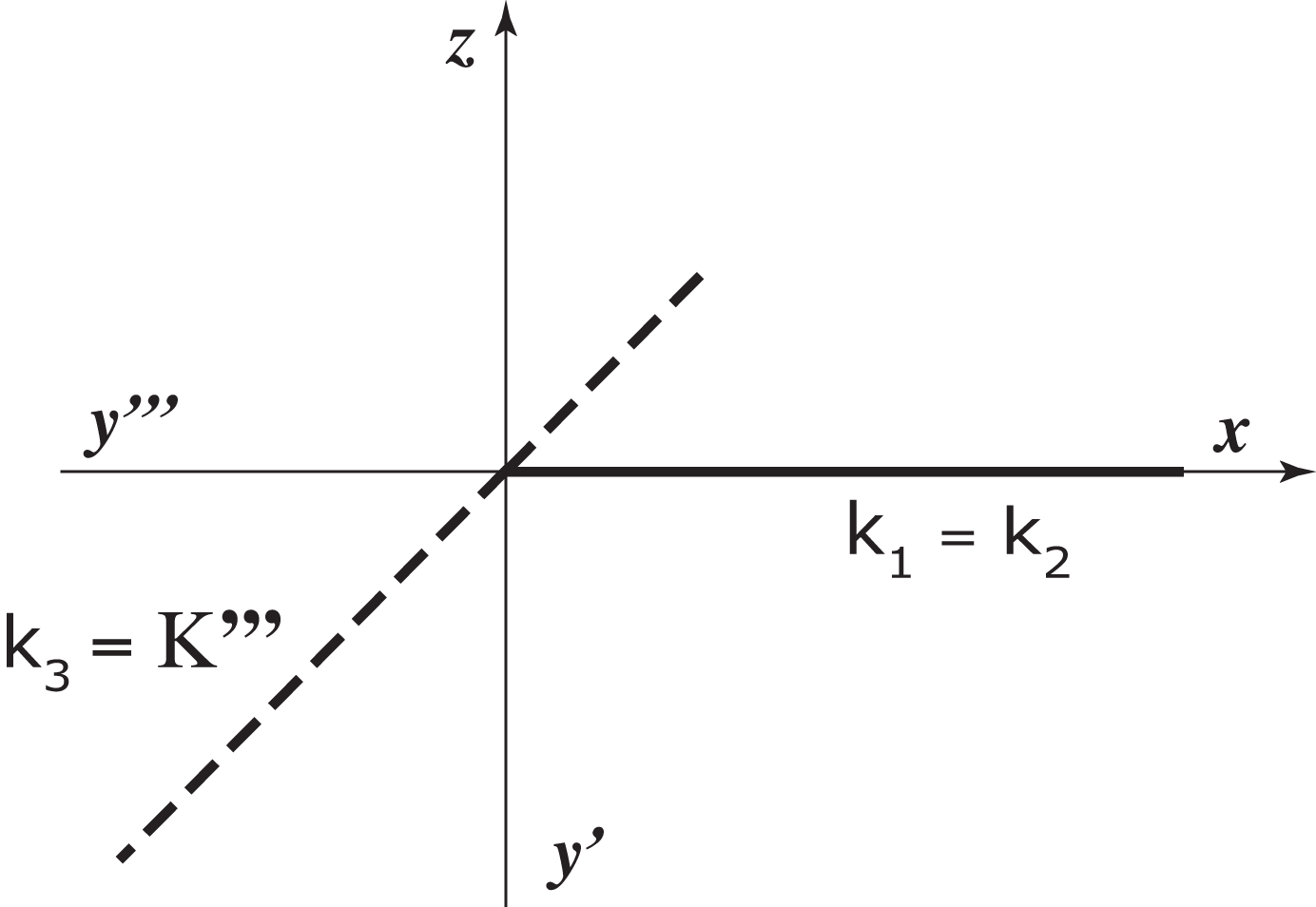Traces of the coincidence plane.

• The plane Ρ(0,0,0) is given by one of its points.
To determine this plane we must know coordinates of another two points, or a line that doesn't pass through the origin. Then the traces can be drawn.

### A line in a plane

 Traces of any line are points contained in Π1 and Π2. Therefore, if a line lies in a plane then and only then its horizontal trace lie on the horizontal trace of the plane and its vertical trace lie on the vertical trace of the plane. And obviously its profile trace lie on the profile trace of the plane. p ⊂ Ρ <=> P1 ∈ r1 & P2 ∈ r2 Construction of the projections of a line in the plane given by its traces.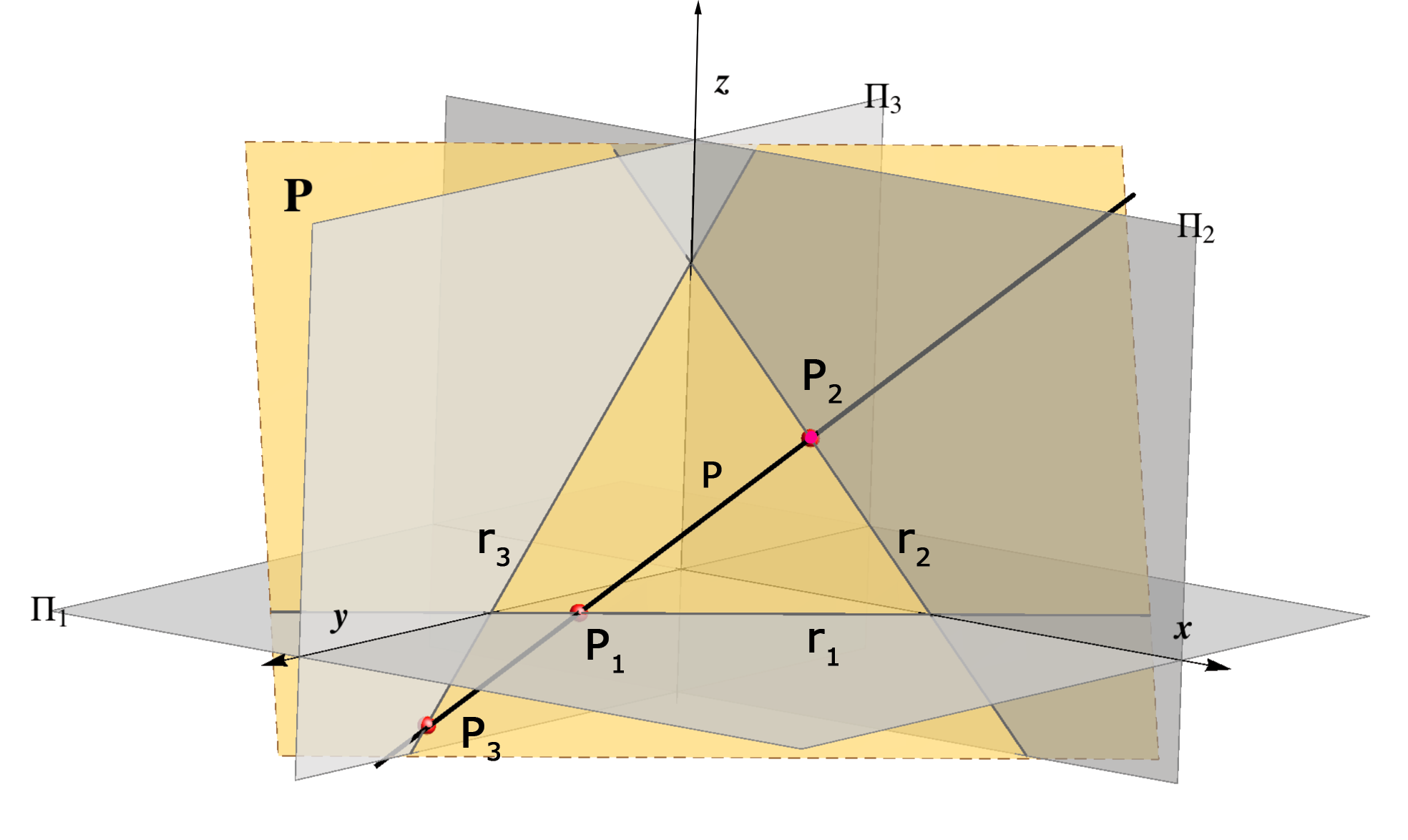Assignment 3: Contruct the traces of the plane that contains the origin if another two points in the plane are given.

### A point in a plane

 Point lies in a plane if and only if the point lies on a line contained in this plane. T ∈ Ρ <=> ∃ p ⊂ Ρ & T ∈ p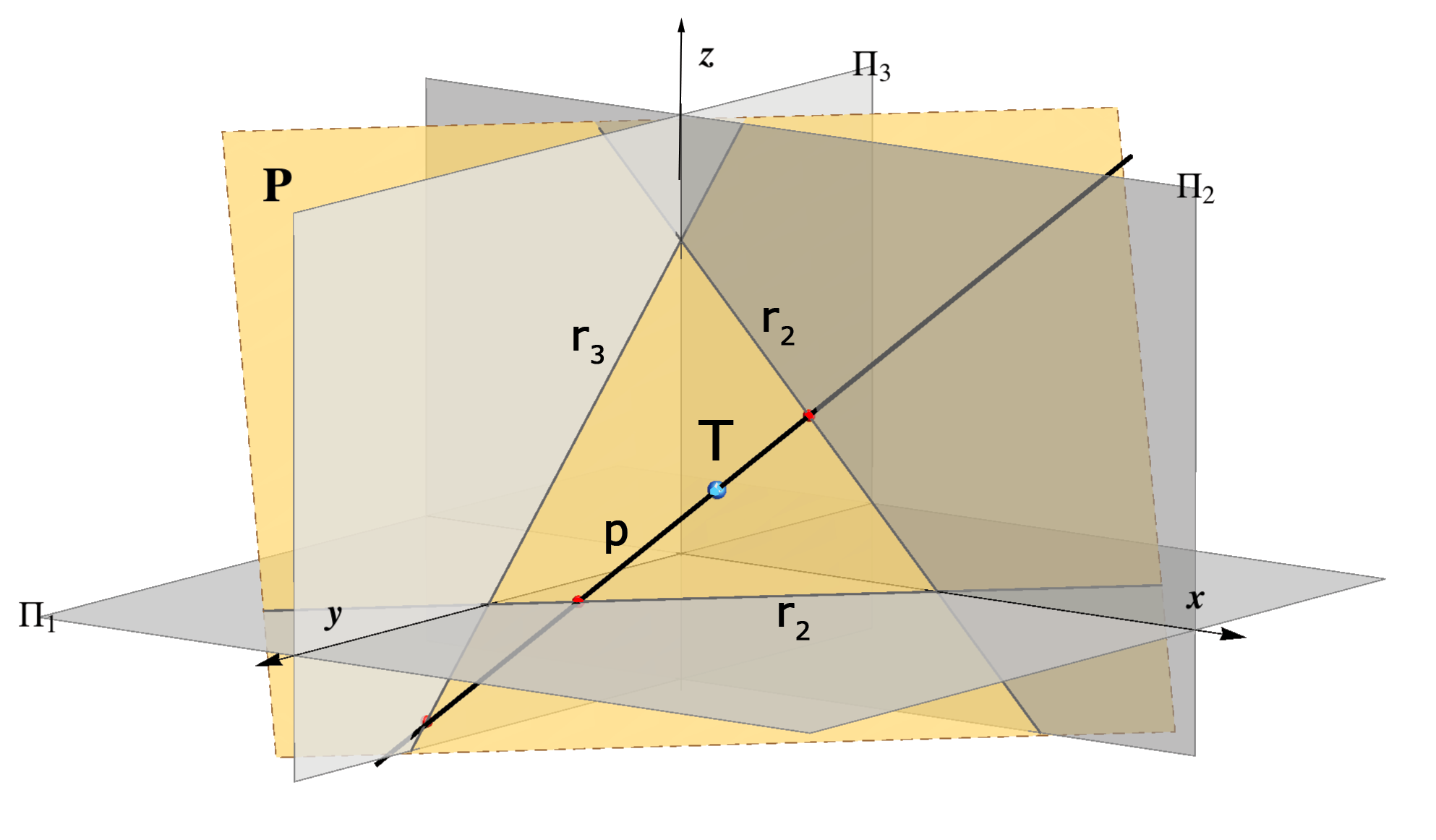Assignment 4: Construct the vertical projection (front view) of the point T(2,2,–), if the point lies in the plane P(4,5,3).

### Determining the traces

It is a simple task to construct the traces of a plane defined by two intersecting lines, by two parallel lines, by a line and a point that doesn't lie on it or by three non-collinear points:
 Plane defined by  parallel lines p and q. Construction is shown in the image on the rightside. Click on the image to start the animation. Describe by words the principle of the construction.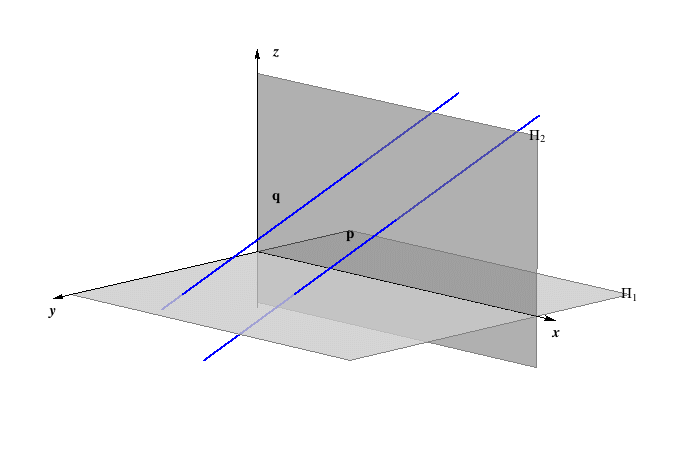A plane defined by  intersecting lines p and q. Construction is shown in the image on the rightside. Click on the image to start the animation. Describe in words the principle of the construction.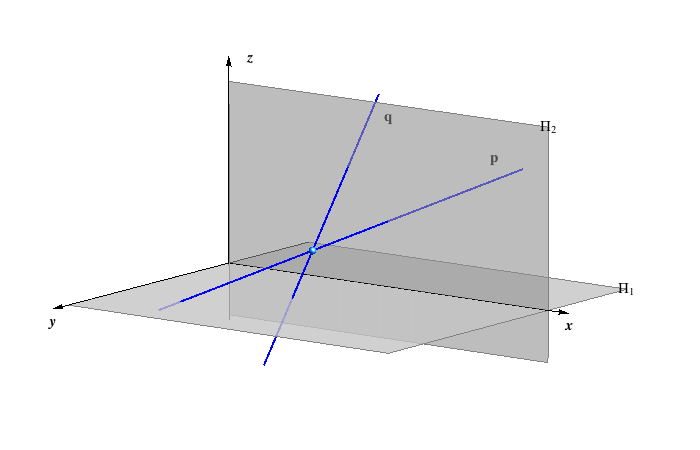• When the plane is defined by the line p and the point P ∉ p or by three non-collinear points  A, B and C, the construction of the traces is reduced to the cases above.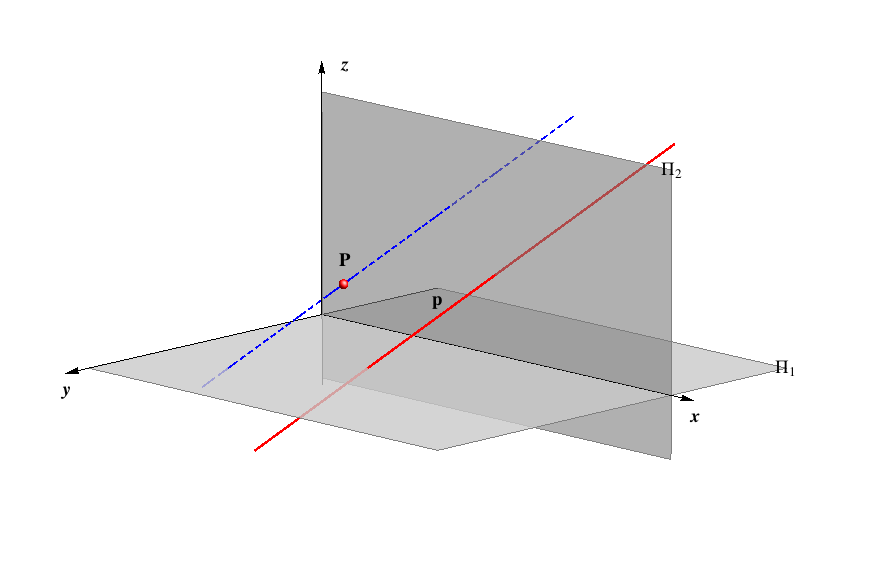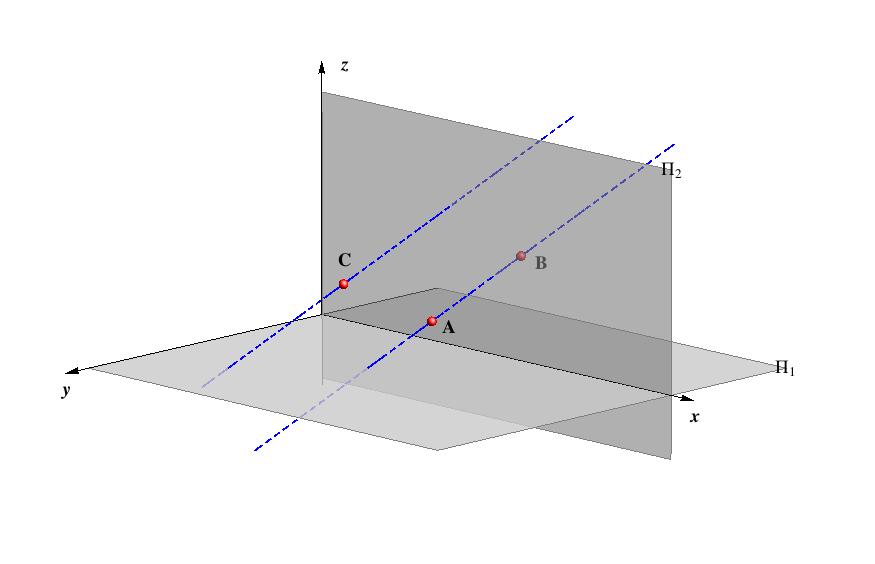### Two planes

Two planes Ρ and Σ can be parallel or they intersect along the line p.

• If the planes Ρ and Σ are parallel, then the corresponding traces are parallel as well, i.e.

Ρ | | Σ=>r1 | | s1 & r2 | | s2.

• If the planes Ρ and Σ intersect, then the traces of their intersecting line p are intersections of corresponding traces of the planes, i.e.

ΡΣ = P1P2,ifP1 = r1s1 & P2 = r2s2.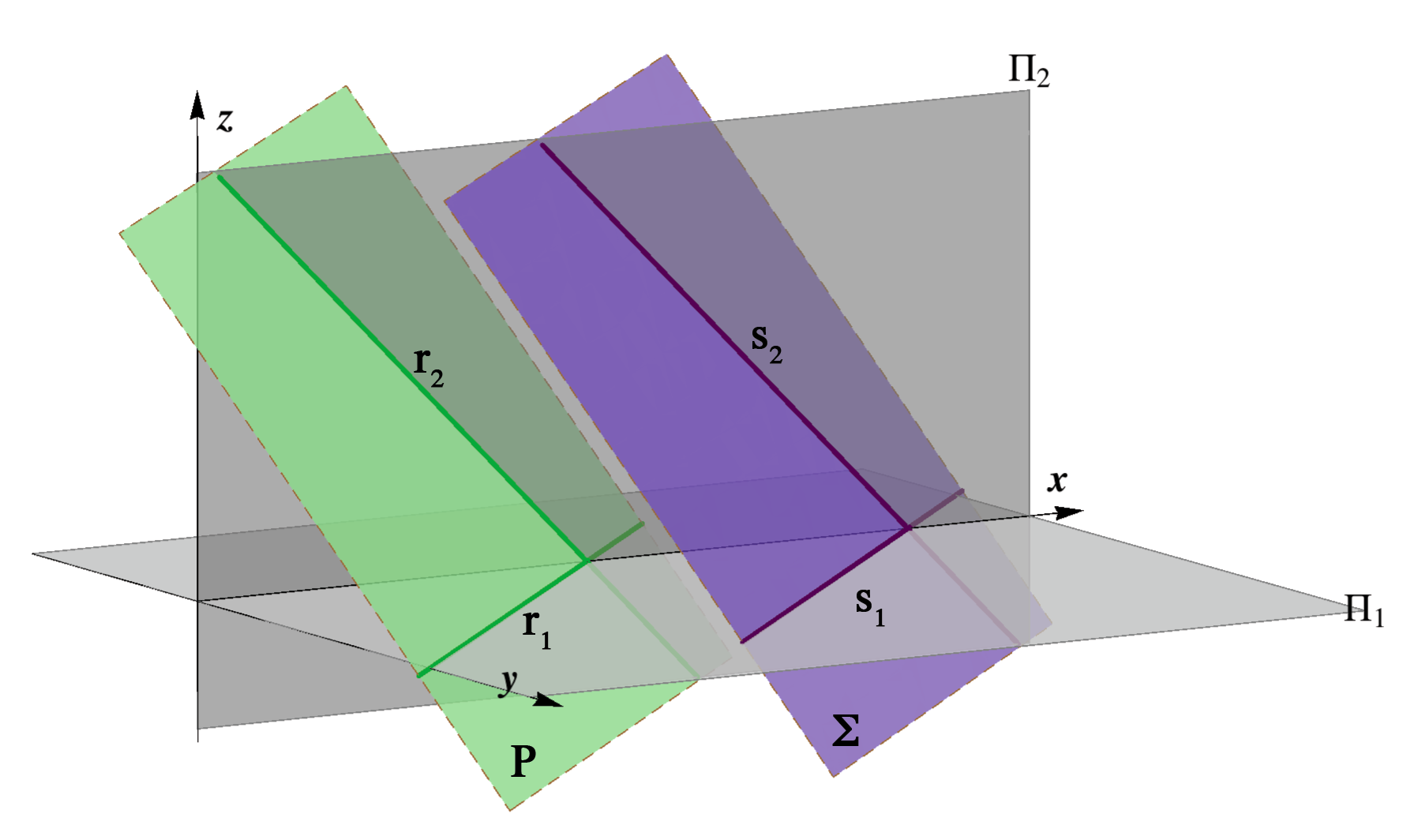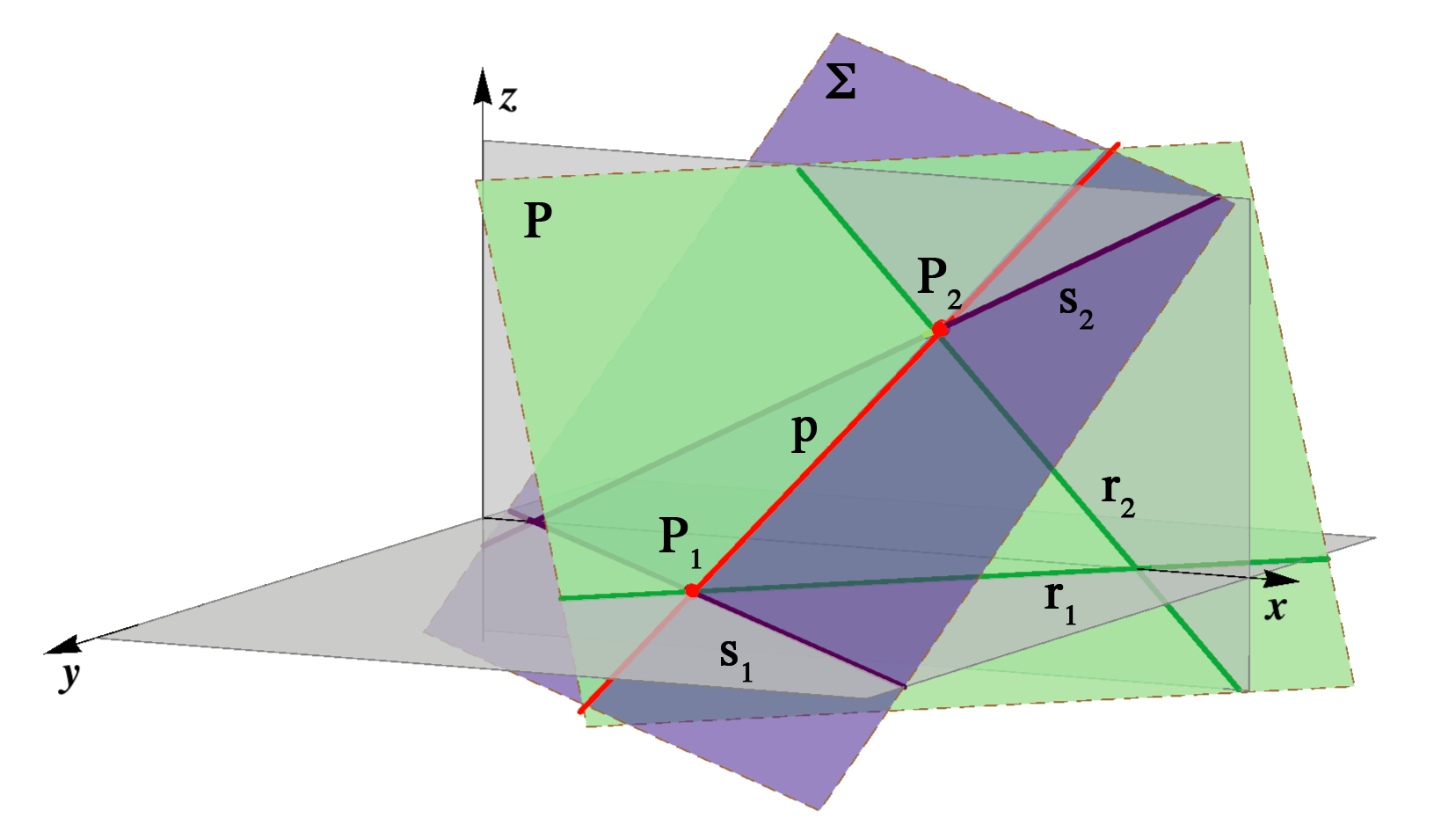Parallel planes. Planes intersect along the line p.

A construction of the planes from the pencil (p).

### Principal lines of a plane

#### Principal lines

• Principal lines are lines in the plane parallel to the projection planes.
They are divided into three groups, depending on the plane they are parallel to:
• Line a is a horizontal principal line of the plane Ρ if a | | Π1, i.e. a is a horizontal line.
Its projections satisfy the following: a' | | r1, a'' | | x, a''' | | y.
• Line b is a vertical principal line of the plane Ρ if b | | Π2, i.e. b is a vertical line.
Its projections satisfy the following: b' | | x, b'' | | r2, b''' | | z.
• Line c is a profile principal line of the plane Ρ if c | | Π3, i.e. c is a profile line.
Its projections satisfy the following: c' | | y, c'' | | z, c''' | | r3.

 a — H principal line b — V principal line c — P principal line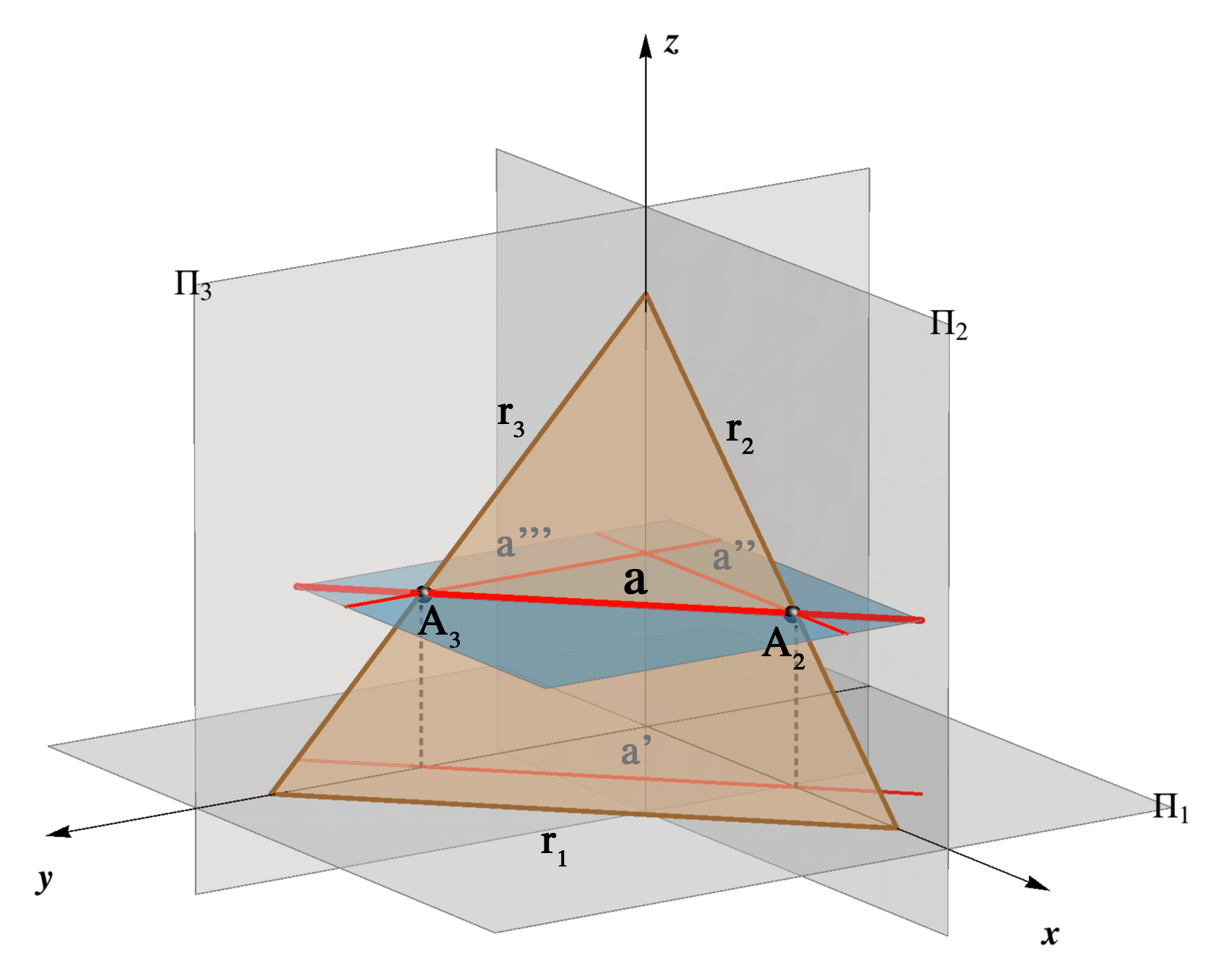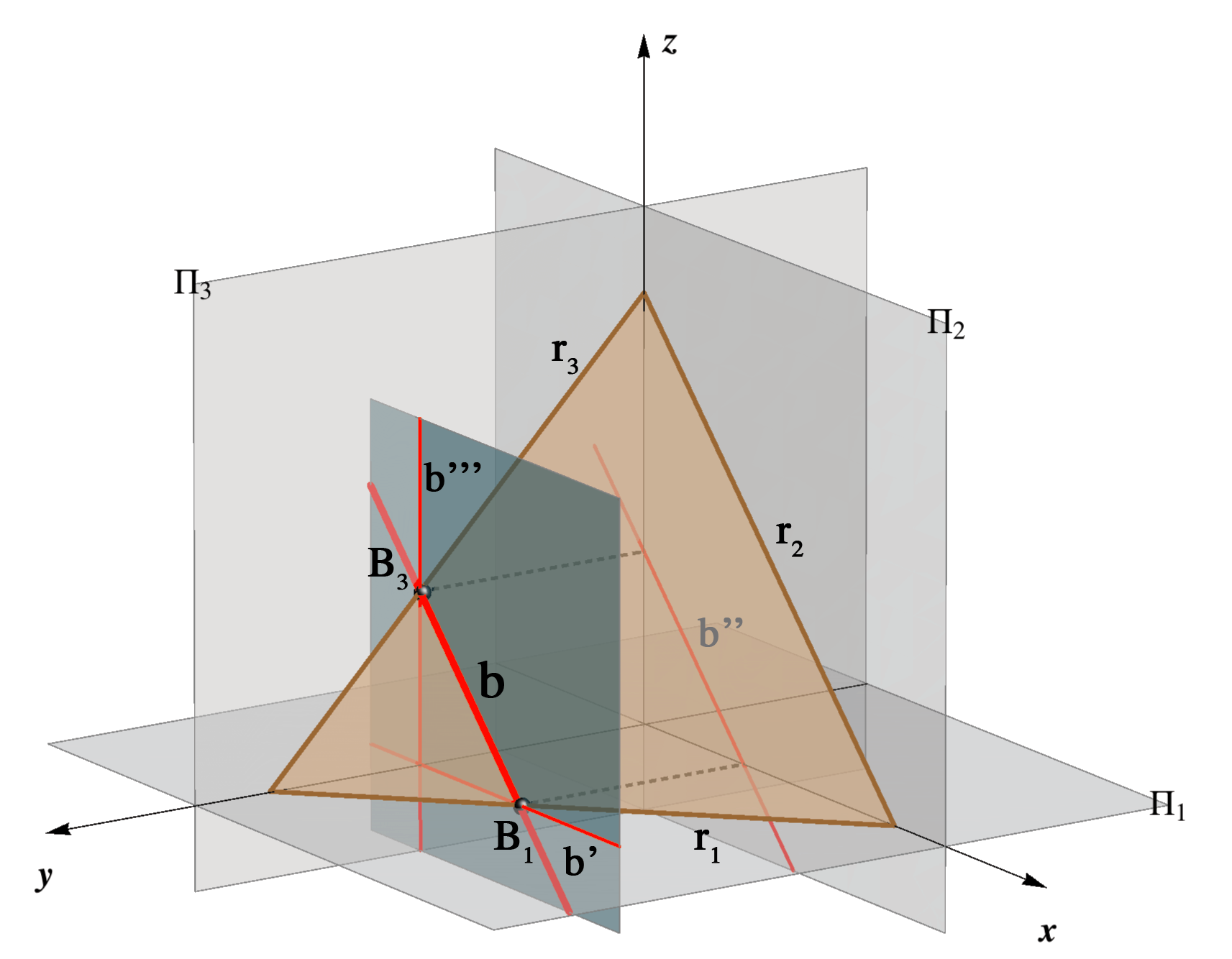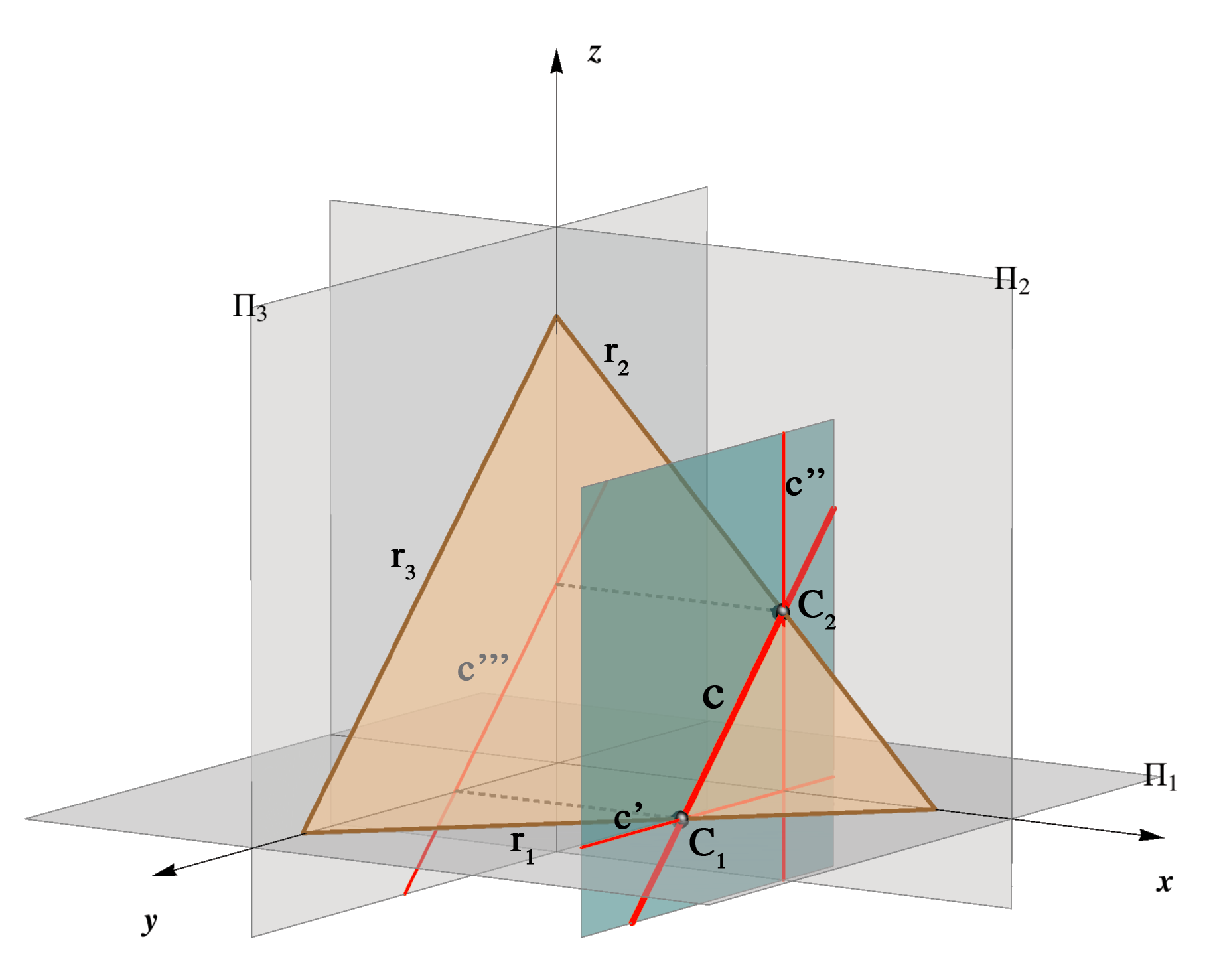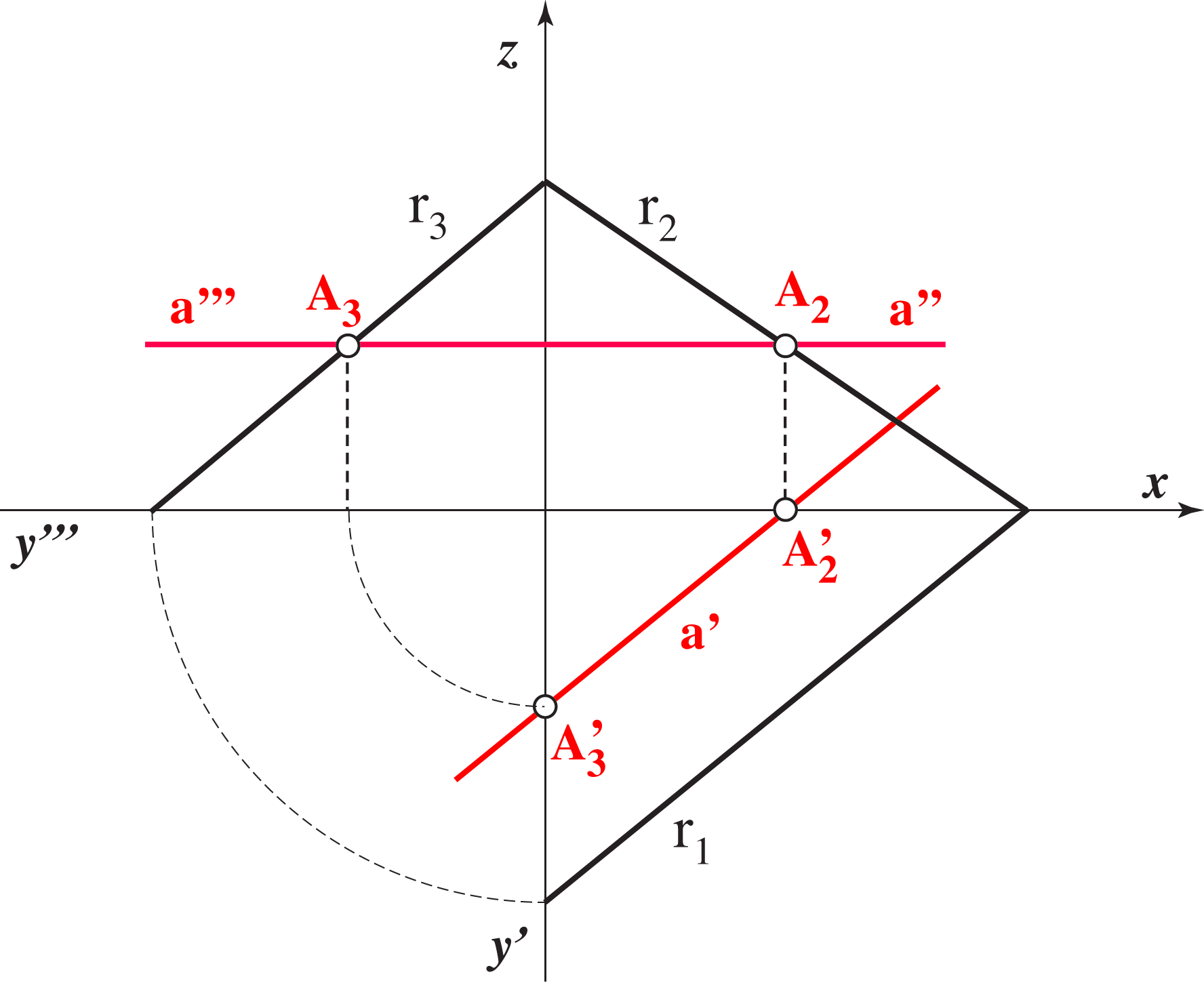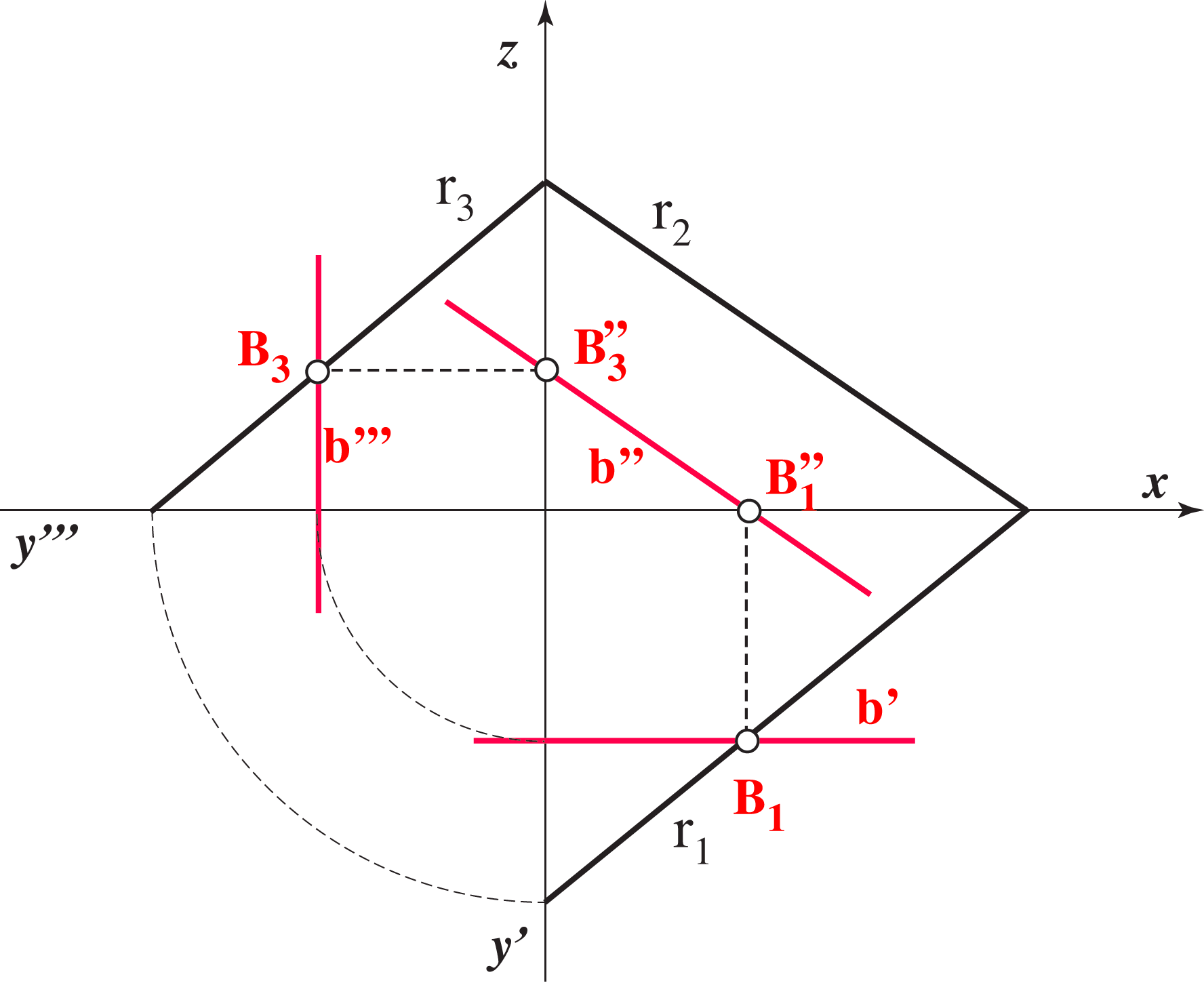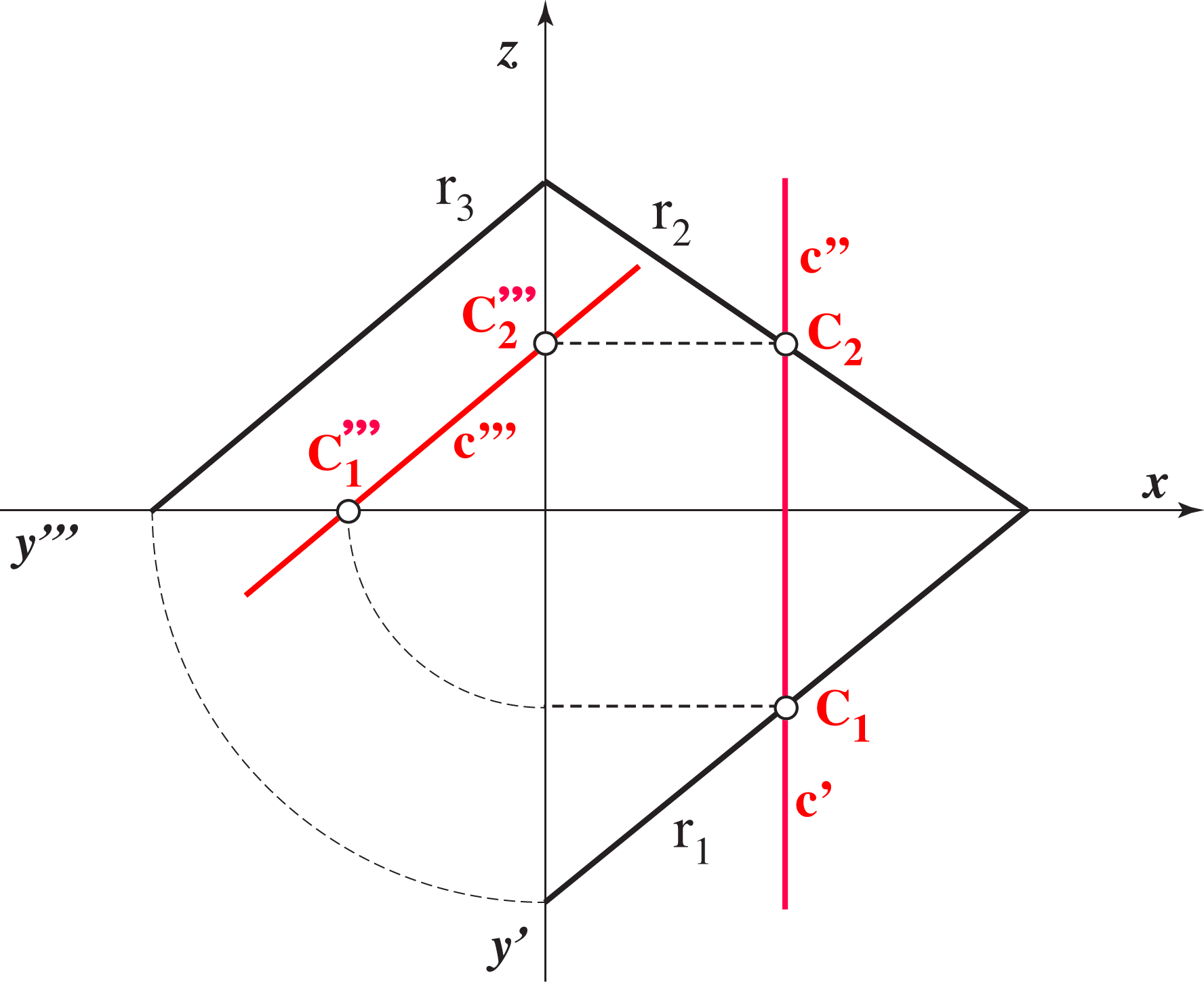A construction of projections of the point in the plane given by its traces.

A construction on the traces of the plane parallel to the given plane that passes through the given point

#### Steepest lines of the plane

• Steepest line of the plane is a line in the plane that is perpendicular to one of its traces.

They are divided into three groups, depending on the trace they are perpendicular to:
• The line a is the 1st steepest line of a plane of the plane Ρ  if it is a line in the plane such that a ⊥ r1.
A horizontal projection of the 1st line of inclination is perpendicular to the horizontal trace of the plane, i.e.  a' ⊥ r1.
• The line b is the 2nd steepest line of a plane of the plane Ρ if bΡ and b ⊥ r2.
A vertical projection of such lines is perpendicular to the vertical trace of the plane, i.e. b'' ⊥ r2.
• The line c is the 3rd steepest line of a plane of the plane Ρ if cΡ and c ⊥ r3.
A profile projection of such lines is perpendicular to the profile trace, i.e. c''' ⊥ r3.

Line p that is 1st steepest line of the plane Ρ is shown in the figures below.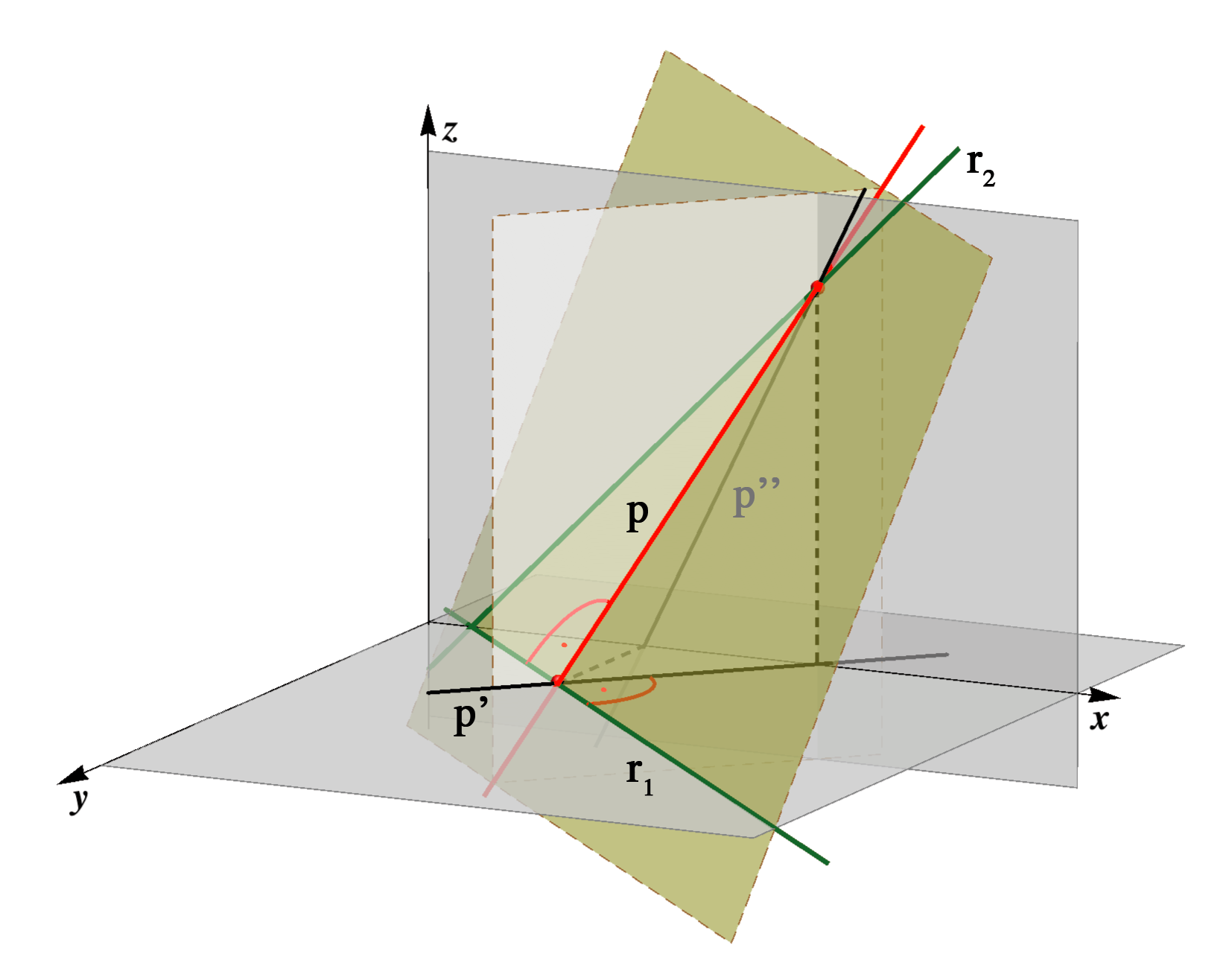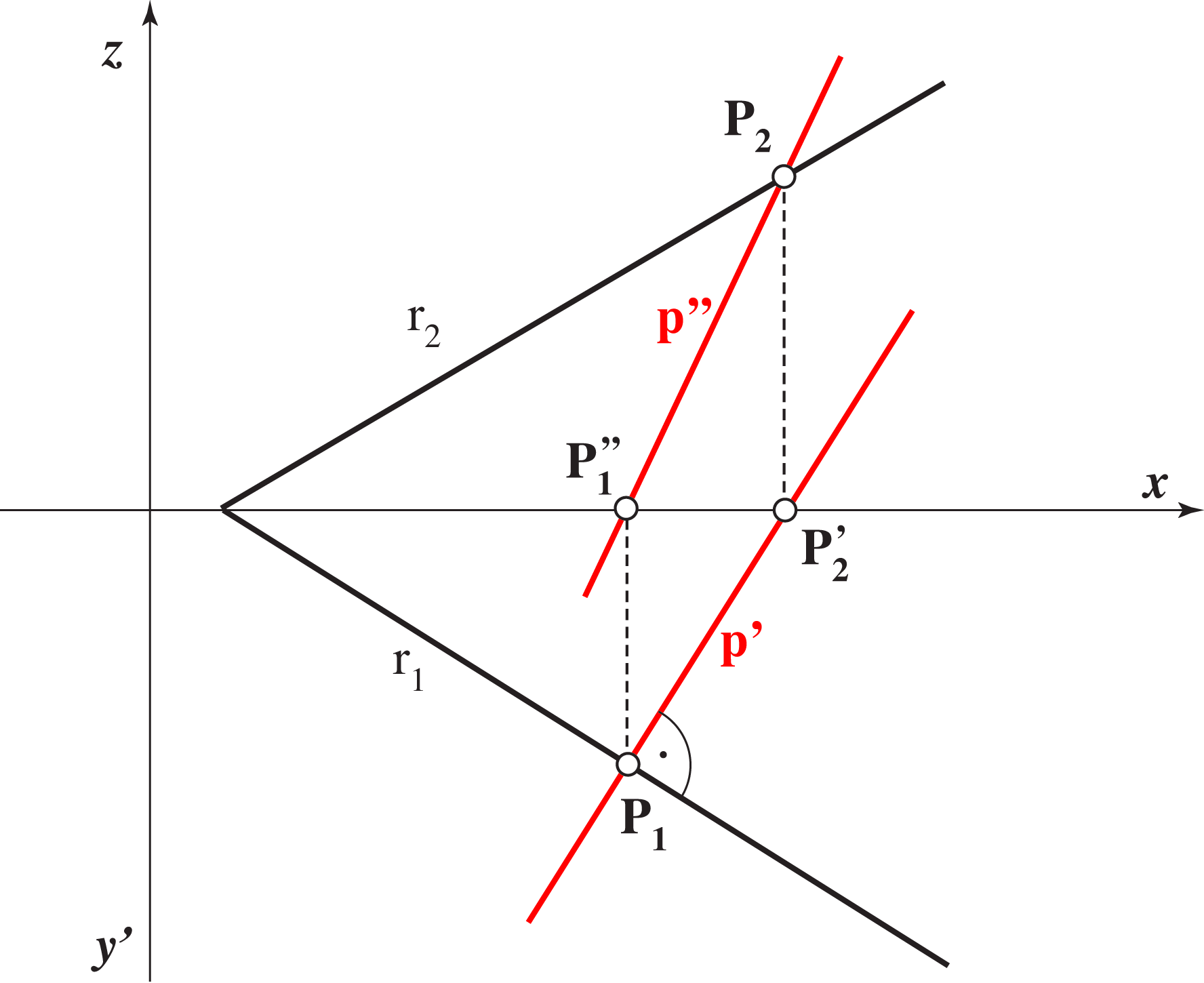#### The angle of inclination

• The angle of inclination of the plane is the angle between the plane and the plane of projection.

There are three angles of inclination of the plane Ρ, depending on the three planes of projection,
1st, 2nd or 3rd angle of inclination denoted by ω1, ω2 or ω3.

The angle between two planes is defined as the angle between two lines, so:
• ω1 = ∠ (a,a'), where a is any 1st steepest line of the plane Ρ.
• ω2 = ∠ ((b,b''), where b  is any 2nd steepest line of the plane Ρ.
• ω3 = ∠ ((c,c'''), where c  is any 3rd steepest line of the plane Ρ.

Images below show the first angle of inclination of the plane Ρ as the angle between one1st steepest line and its horizontal projection. There is also the construction of the true size of that angle.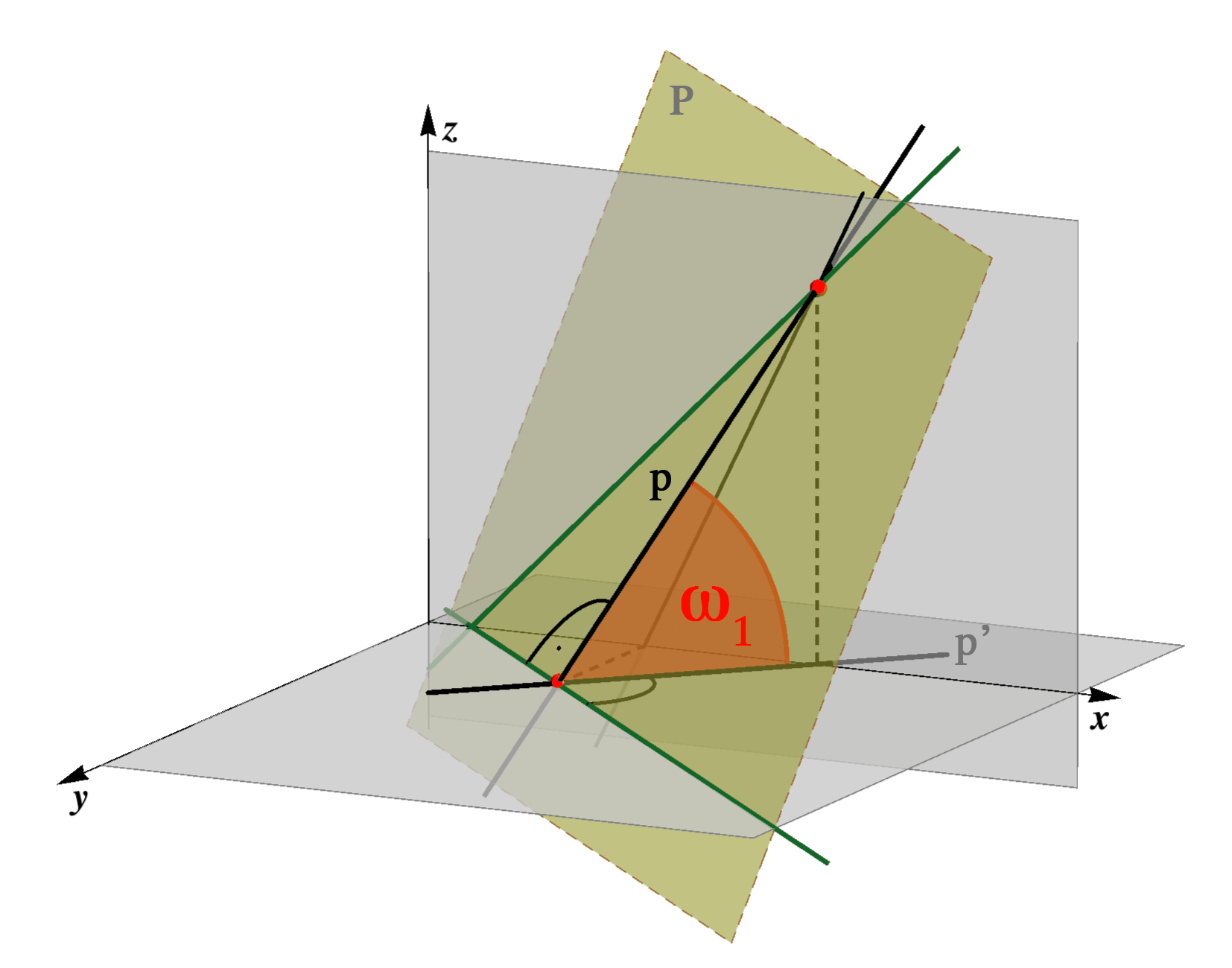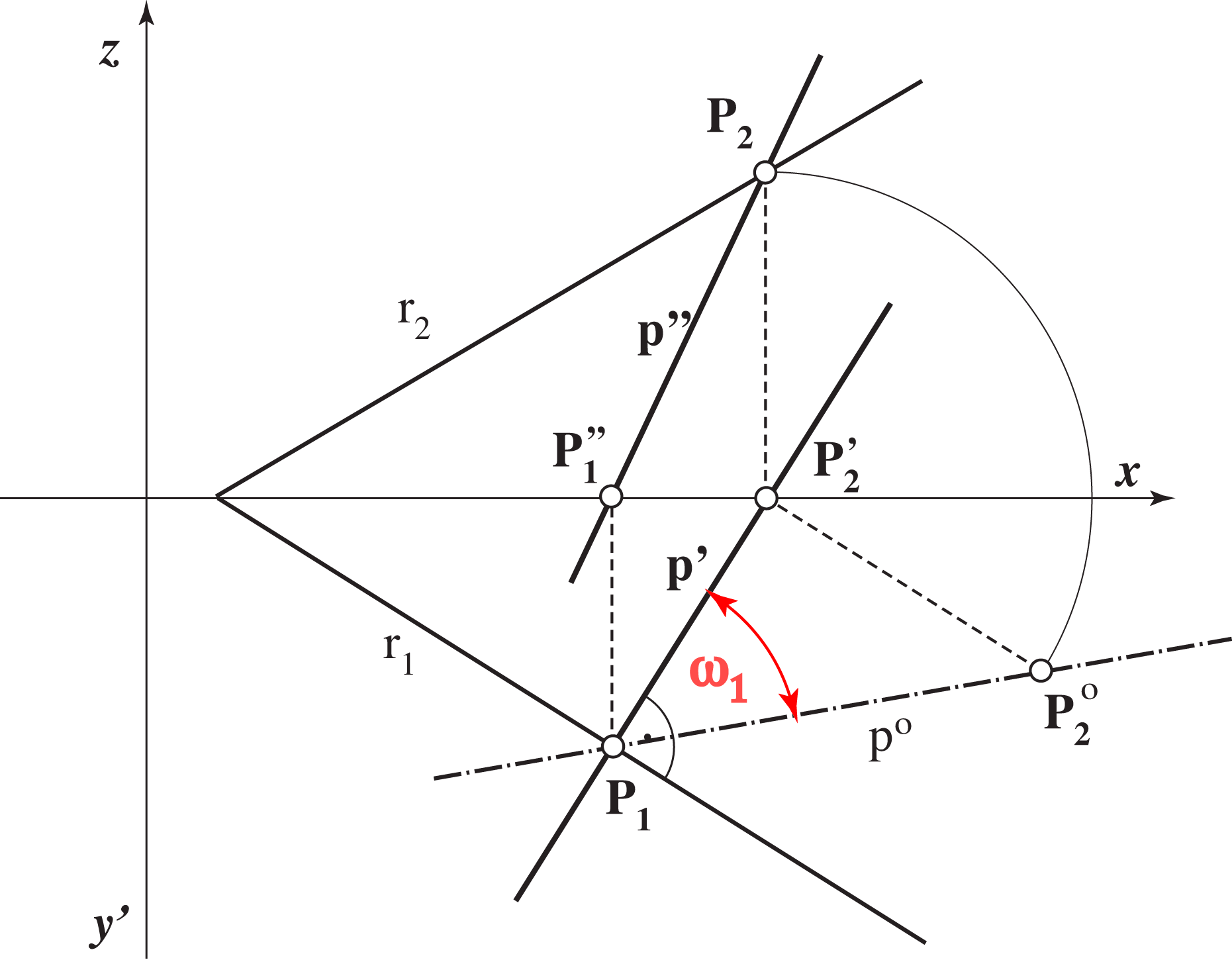Created by Sonja Gorjanc, translated by Helena Halas and Iva Kodrnja - 3DGeomTeh - Developing project of the University of Zagreb